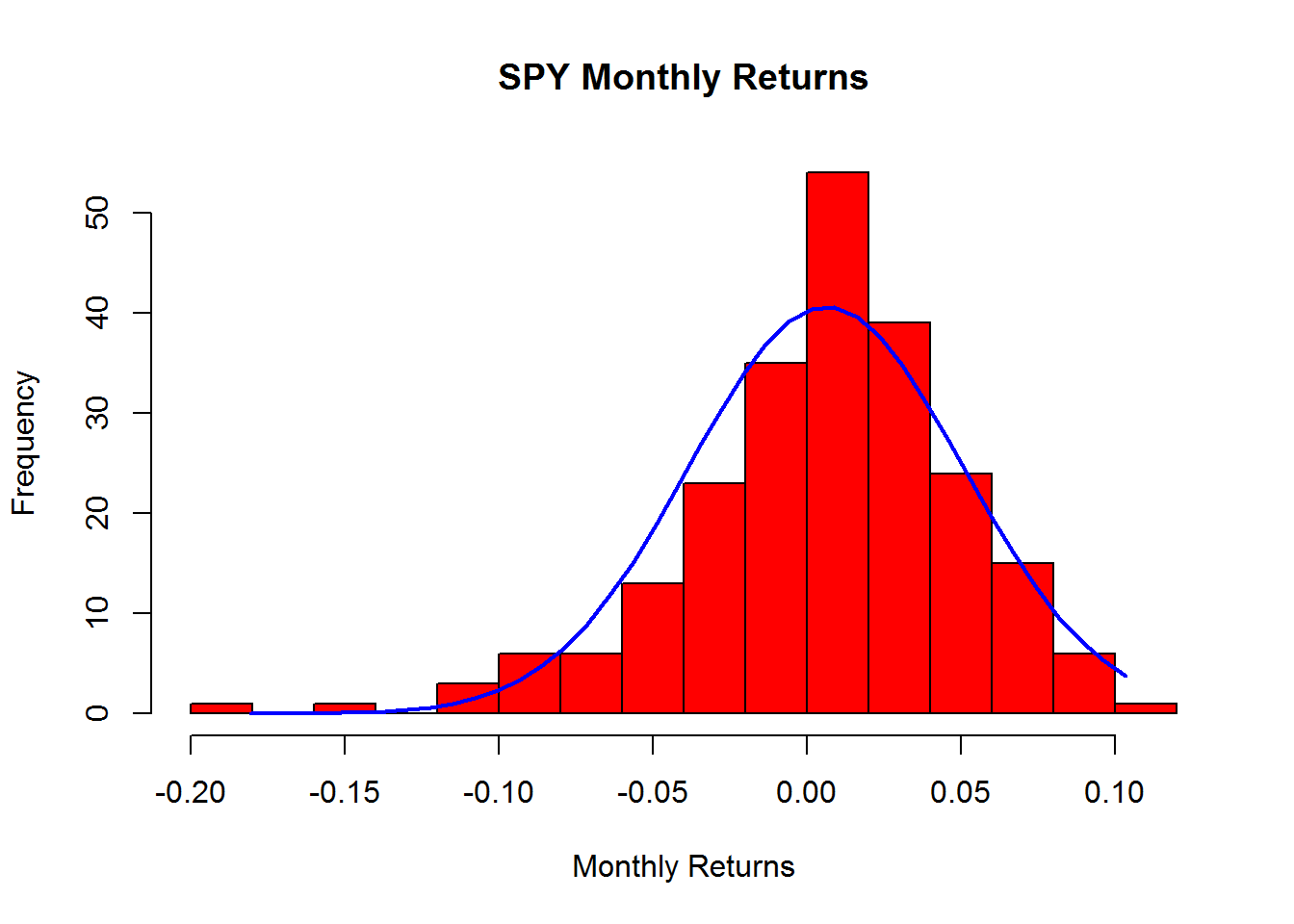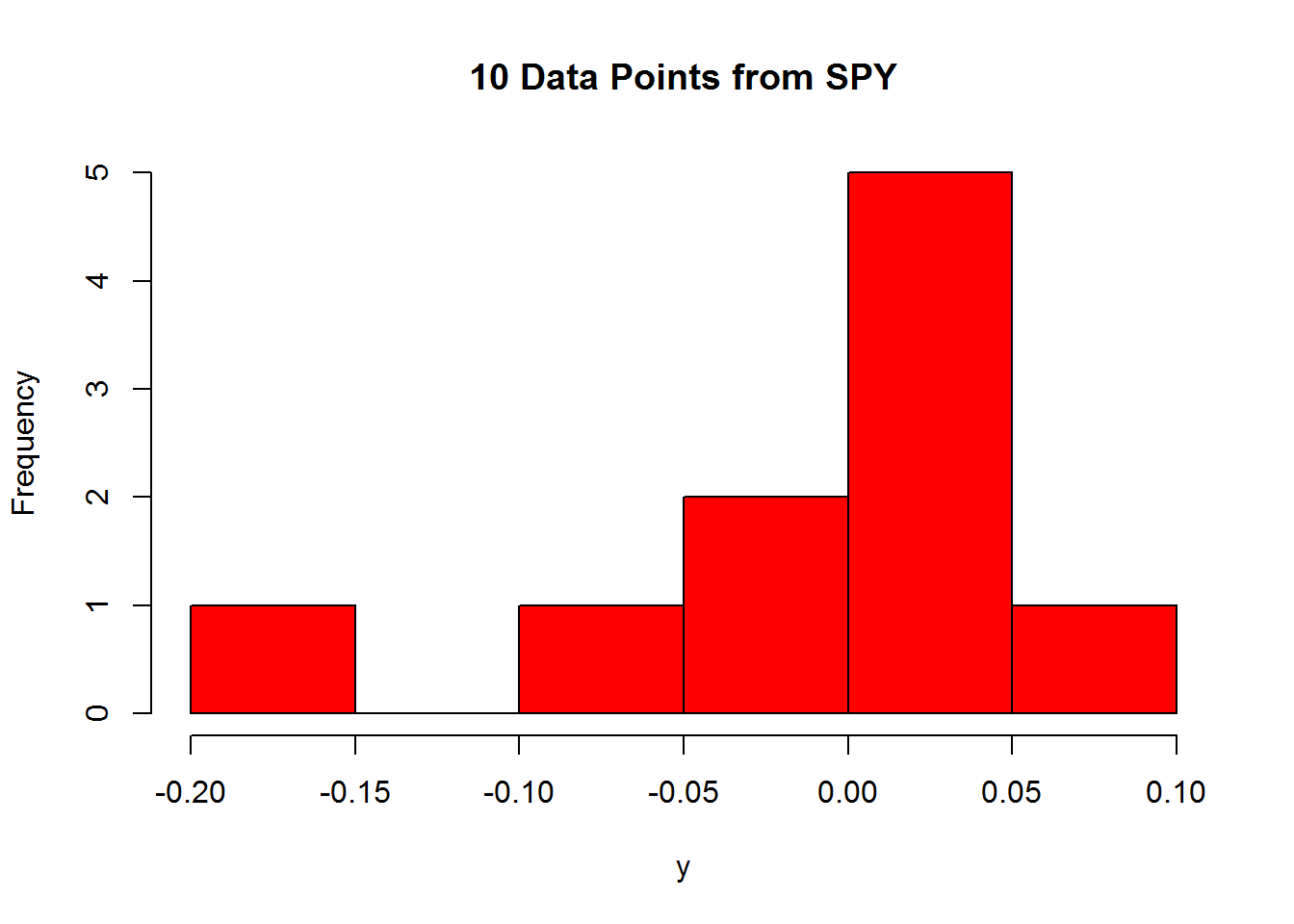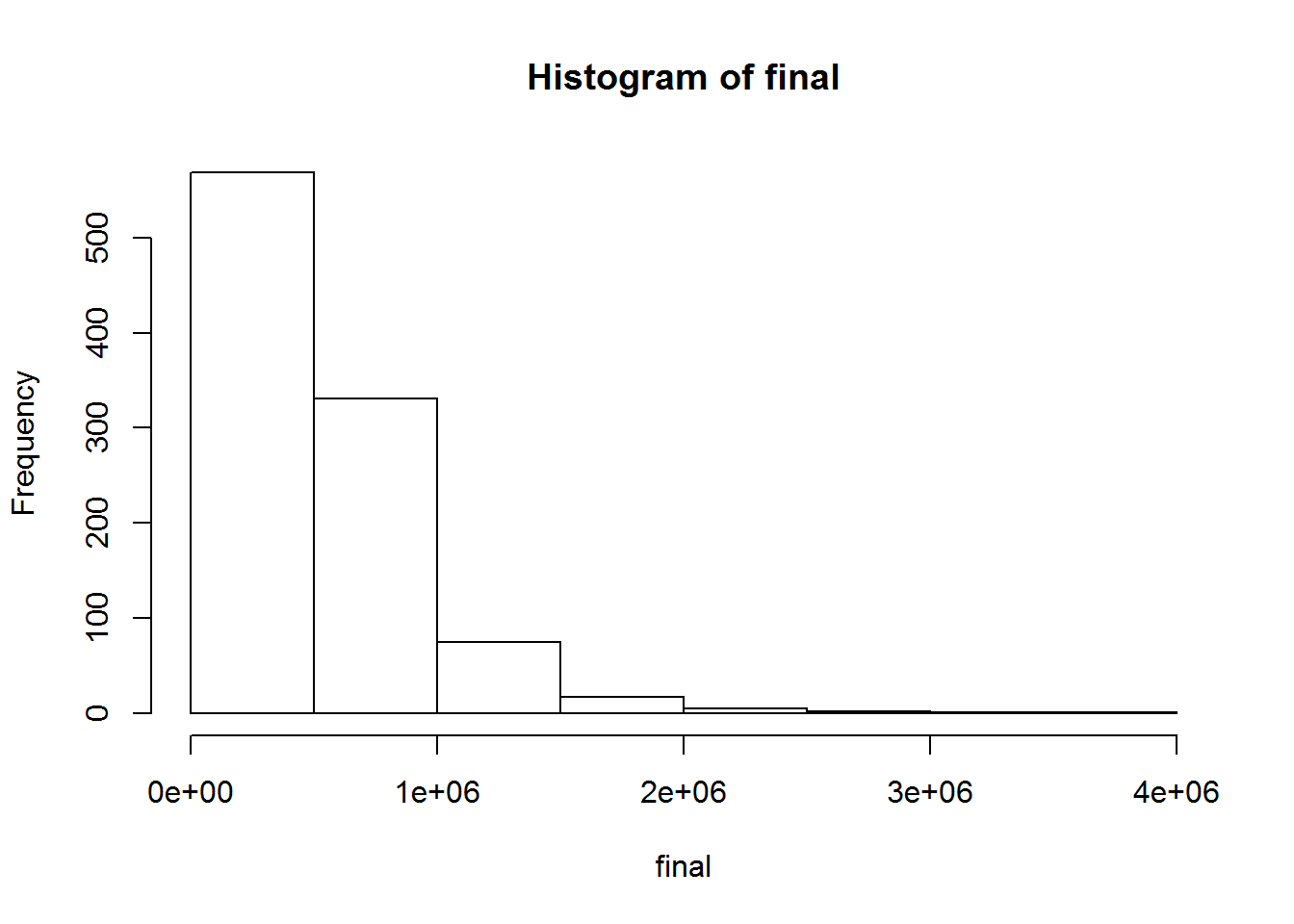The goal of this lesson is to demonstrate how to conduct Monte Carlo simulation by sampling actual empirical data. In the last few lessons, we have either tried to guess a distribution (often using the triangle distribution) or have assumed that given data, such as mutual fund returns, is close enough to a normal (or some other) distribution and that we can therefore generate numbers randomly from a normal (or other) statistical distribution. There are times, however, when it is best to conduct a Monte Carlo simulation by randomly sampling from empirical data. In other words, rather than sampling from an approximation (the blue line below), why don’t we sample from actual empirical data, as is represented by the underlying red histogram.In order to do this, you will need plenty of empirical data. You definitely don’t want to go down the sampling road if you only have a handful of observations. Sampling from a handful of observations will tend to skew your results heavily toward the few observations that you have. These few observations may not fully represent the underlying distribution. For example, let’s say I only have 10 observations of the underlying data for the SPY monthly returns seen above.You see here that with only 10 data points, that this is not necessarily representative of the original distribution, and if I were to randomly select one number from these 10, my results would be skewed toward these numbers. If we have enough data, however, than sampling from this empirical data can provide a better representation.

Sampling is also possible when the data isn’t approximated by any known statistical distribution. If your data can’t be approximated by a statistical distribution, then you are forced to sample from an empirical distribution.

First, let’s show how the sample command works. If I want to sample 10 numbers from the SPY data, I would use the following command

x<-c(1,7,9.2,15,25,4,2,7,9,4,6,4,9.2)
sample(x,size=5,replace=TRUE)
##  25  4  7  4 25

Note that this says to get a sample of size 5 from the vector x, and that the sampling is done with replacement (meaning that you could sample the same number twice). Note that this will randomly grab five actual numbers from our vector.

Below we will illustrate how to use this concept for Monte Carlo simulation with the SPY (S&P 500) mutual fund. In this case we will invest \$400 every month into this mutual fund, and we want to see what it will be at the end of 30 years (360 months). Rather than pull an interest rate from a random normal distribution, however, we will sample a rate from actual SPY rates. This is done below in a nested for loop

final<-NULL
for(i in 1:1000){
total<-NULL
rate<-sample(fund.returns,size=1,replace=TRUE)
total<-400*(1+rate)
for(j in 2:360){
rate<-sample(fund.returns,size=1,replace=TRUE)
total[j]<-(400+total[j-1])*(1+rate)
}
final[i]<-total
}

This provides a vector (final) that is 1000 long and is the final amount in the account after 30 years, or 360 months. This is a better approximation that our earlier attempts.

Here’s the histogram of the output:

hist(final)and here’s a summary of the output:

summary(final)
##    Min. 1st Qu.  Median    Mean 3rd Qu.    Max.
##   73800  301000  443000  547000  675000 3610000